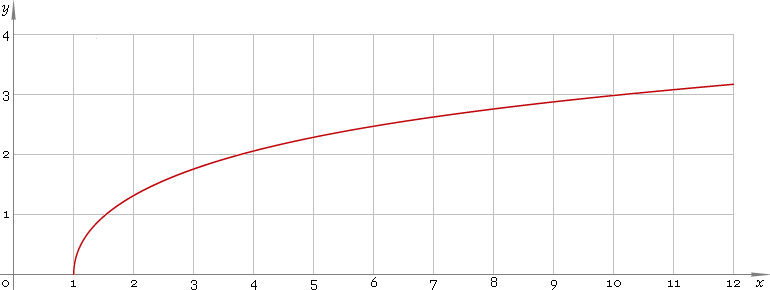The Art of Mathematics

# arcosh or arch — arc-hyperbolic cosine function

## 1. Definition

Arc-hyperbolic cosine is inverse of the hyperbolic cosine function.

arcoshxcoshinvx

With the help of natural logarithm it can be represented as:

arcoshxln[x + √(x2 − 1)]

## 2. Graph

Arc-hyperbolic cosine is monotone function defined in the range [1, +∞). Its graph is depicted below — fig. 1.Fig. 1. Graph of the arc-hyperbolic cosine function y = arcoshx.

Function codomain is non-negative part of real axis: [0, +∞).

## 3. Identities

Reciprocal argument:

arcosh(1/x) = arsechx

Sum and difference:

arcoshx + arcoshy = arcosh{xy + √[(x2 − 1)(y2 − 1)]}
arcoshx − arcoshy = arcosh{xy − √[(x2 − 1)(y2 − 1)]}
arsinhx + arcoshy = arsinh{xy + √[(x2 + 1)(y2 − 1)]} = arcosh[y√(x2 + 1) + x√(y2 − 1)]

## 4. Derivative and indefinite integral

Arc-hyperbolic cosine derivative:

arcosh′x = 1 /√(x2 − 1)

Indefinite integral of the arc-hyperbolic cosine:

∫ arcoshx dx = x arcoshx − √(x2 − 1) + C

where C is an arbitrary constant.

## 5. How to use

To calculate arc-hyperbolic cosine of the number:

``arcosh(2);``

To get arc-hyperbolic cosine of the complex number:

``arcosh(2+i);``

To get arc-hyperbolic cosine of the current result:

``arcosh(rslt);``

To get arc-hyperbolic cosine of the number z in calculator memory:

``arcosh(mem[z]);``

## 6. Support

Arc-hyperbolic cosine of the real argument is supported in free version of the Librow calculator.

Arc-hyperbolic cosine of the complex argument is supported in professional version of the Librow calculator.